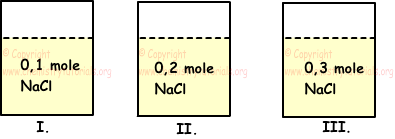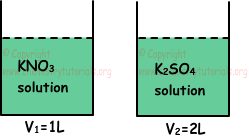EN | ES | DE | NL | RU

## Properties of Solutions and Solution Calculations

Properties of Solutions

• Boiling point, freezing point, vapor pressure, and properties like density of solutions are different from properties of pure solvent. For example, water boils at 100 oC, on the contrary salt water solution boils  above 100 0C.
• If dissolved matter in water is not volatile, they prevent vaporization of water and as a result boiling point of water increases and freezing point of water decreases. In winter, salt is poured on road to decrease freezing point of water.
• In a solution, increasing boiling point is directly proportional to molar concentration of particles in solution.
• In liquid solutions, decreasing of freezing points and vapor pressure is inversely proportional to molar concentration of particles in solution.
• Conductivity of electricity is directly proportional to molar concentrations of ions in solutions. For example, alcohol and sugar do not conduct electricity.

Example: There are equal amounts of water in following tanks. If we dissolve following solutes in these tanks; find relation between electrical conductivity of these solutions.

I. 1 mol NaCl

II. 2 mol sugar

III. 1 mol Al(NO3)3Solution:

Electrical conductivity of solutions depends on molar ion concentration of solution. Now we find ion concentration of solutions in each tank.

I. NaCl(s) → Na+(aq) + Cl-(aq)

1 mol        1 mol      1 mol

There are 2 mole ion in first solution.

II. Since sugar does molecular solvation, there is no ion in the solution. So, it does not conduct electricity.

III. Al(NO3)3(s) → Al+3 + 3NO3-1

1 mol         1 mol   3 mol

There are 4 mol ion in this solution.

Volumes of solutions are equal to each other thus relation between electrical conductivity of solutions becomes;

III>I>II

Solution Calculations

Example: If we prepare 3 solutions under 1 atm pressure using 1 L water and 0,1 mol NaCl, 0,2 mol NaCl, 0,3 mol NaCl for each solution, compare boiling point, freezing point and vapor pressures of these solutions.Solution:

NaCl(s) → Na+(aq) + Cl-(aq)

1 mol NaCl gives 1 mol Na+ and 1 mol Cl- total 2 mol ions.

In first container; 0,1 NaCl gives 0,2 mol ion

In second container; 0,2 NaCl gives 0,4 mol ion

In third container; 0,3 NaCl gives 0,6 mol ion

Boiling points of solutions related to amount of solute in solution. Increasing in the amount of solute increase boiling point of the solution. Relation between boiling points of solution;

III>II>I

If boiling point of solution is high, then vapor pressure of it is low. Thus relation between vapor pressures of solutions becomes;

I>II>III

If boiling point of solution is high, then freezing point of it is low. Thus relation between freezing points of solutions becomes;

I>II>III

Example: Compare boiling points of following solutions;

I. Unsaturated X solid-water solution

II. Saturated X solid-water solution

III. Supersaturated X solid-water solution

Solution:

As amount of solute increases in solution,, boiling point also increases. Thus, III has largest solute in it then II and finally I. Relation between them becomes;

III>II>I

Example: Containers given below includes solutions under same temperature and pressure. If molar concentration of K+ ion in both containers are equal, which ones of the following statements are true for these solutions?

I. Number of moles of solute matters are equal.

II. Vapor pressure of first container is higher than second container.

III. Boiling point of first solution is lower than second solution.Solution:

Assume that, molar concentration of  [K+]=2 M;

I.

1 mol KNO3 includes 1 mol K+, so 2 M K+ ion comes from 2 M KNO3 .

1 mol K2SO4 includes 2 mol K+, so 2 M K+ ion comes from 1 M K2SO4. Thus, moles of dissolved KNO3 and K2SO4;

nKNO3=V1.M1=1.2=2 mol

nK2SO4=V2.M2=2.1=2 mol

So, number of moles of dissolved matters are equal, I is true.

II. 2 M KNO3 gives 4 M ion and 1 M K2SO4 gives 3 M ion. Vapor pressure is inversely proportional to molar concentration of particles in solution. Thus, vapor pressure of second container is higher than first container. II is false.

III. Boiling point is directly proportional to molar concentration of particles in solution, thus solution in first container has higher boiling point than solution in second container. III is false.

Solutions Exams and  Problem Solutions

The Original Author:

Tags: Solutionns Cheat Sheet |